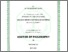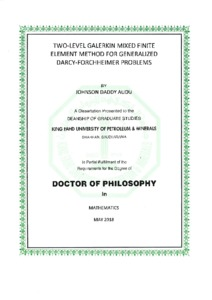# TWO-LEVEL GALERKIN MIXED FINITE ELEMENT METHOD FOR GENERALIZED DARCY-FORCHHEIMER PROBLEMS

TWO-LEVEL GALERKIN MIXED FINITE ELEMENT METHOD FOR GENERALIZED DARCY-FORCHHEIMER PROBLEMS. PhD thesis, King Fahd University of Petroleum and Minerals.Preview
PDF
TWO_LEVEL_GALERKIN_MIXED_FINITE_ELEMENT_METHOD_FOR_DARCY-FORCHHEIMER_PROBLEMS.pdf

We consider the generalized Darcy-Forchheimer equation $$\frac{\mu}{\rho}\boldsymbol{K}^{-1}\boldsymbol{u}+ \frac{\beta}{\rho}|\boldsymbol{u}|^{m-1}\boldsymbol{u} + \bigtriangledown p = \boldsymbol{f} \qquad \mbox{in}~~\Omega,~~ m\in(1,\ \ 2],$$ where $\Omega$ an open bounded domain of $\mathbb{R}^d$ $(d=2,3)$ , $\boldsymbol{u}$ is the velocity, $p$ is the pressure and $\boldsymbol{f}$ is a given function. The parameters $\rho$, $\mu$, and $\beta$ represent the density, viscosity and dynamic viscosity respectively while $\boldsymbol{K}$ is the permeability tensor. This equation serves as a model for many high velocity flows in porous media, most especially for gas reservoirs and hydrodynamic flows. Under some mild regularity assumptions on the boundary and interior data, we establish the well-posedness of the model. For any $m\in (1, 2]$, we consider mixed finite element approximations of the model using piecewise constant and piecewise linear mixed elements; $[L^{m}(\Omega)]^d$ and $[L^{\frac{m+1}{m}}(\Omega)]^d$ error estimates of the velocity and pressure respectively are of order $O(h)$, where $h$ is the mesh size. For the case $m=2$, we propose and analyze a two-level method for the model. In this case, a priori error estimates are obtained in $[L^2(\Omega)]^d$ and $[L^\frac{3}{2}(\Omega)]^d$ norms respectively. We deduce from these estimates that the coarse and fine mesh are related by $h=O(H^2)$, where $h$ and $H$ are the fine and coarse mesh sizes respectively. The efficiency and accuracy of the method were computationally examined. Numerical results show that the two-level Galerkin mixed finite element method is computationally cost-effective than standard Galerkin mixed finite element and the rate of convergence also agrees with the theoretical results.
We consider the generalized Darcy-Forchheimer equation $$\frac{\mu}{\rho}\boldsymbol{K}^{-1}\boldsymbol{u}+ \frac{\beta}{\rho}|\boldsymbol{u}|^{m-1}\boldsymbol{u} + \bigtriangledown p = \boldsymbol{f} \qquad \mbox{in}~~\Omega,~~ m\in(1,\ \ 2],$$ where $\Omega$ an open bounded domain of $\mathbb{R}^d$ $(d=2,3)$ , $\boldsymbol{u}$ is the velocity, $p$ is the pressure and $\boldsymbol{f}$ is a given function. The parameters $\rho$, $\mu$, and $\beta$ represent the density, viscosity and dynamic viscosity respectively while $\boldsymbol{K}$ is the permeability tensor. This equation serves as a model for many high velocity flows in porous media, most especially for gas reservoirs and hydrodynamic flows. Under some mild regularity assumptions on the boundary and interior data, we establish the well-posedness of the model. For any $m\in (1, 2]$, we consider mixed finite element approximations of the model using piecewise constant and piecewise linear mixed elements; $[L^{m}(\Omega)]^d$ and $[L^{\frac{m+1}{m}}(\Omega)]^d$ error estimates of the velocity and pressure respectively are of order $O(h)$, where $h$ is the mesh size. For the case $m=2$, we propose and analyze a two-level method for the model. In this case, a priori error estimates are obtained in $[L^2(\Omega)]^d$ and $[L^\frac{3}{2}(\Omega)]^d$ norms respectively. We deduce from these estimates that the coarse and fine mesh are related by $h=O(H^2)$, where $h$ and $H$ are the fine and coarse mesh sizes respectively. The efficiency and accuracy of the method were computationally examined. Numerical results show that the two-level Galerkin mixed finite element method is computationally cost-effective than standard Galerkin mixed finite element and the rate of convergence also agrees with the theoretical results.